Hans Walser, 

Star of David Theorem Extended

# 1        The small Star of David

We chose a number in the Pascal Triangle and its six immediate neighbors (Fig. 1). Now we connect the six neighbors by two equilateral triangles, forming a Star of David.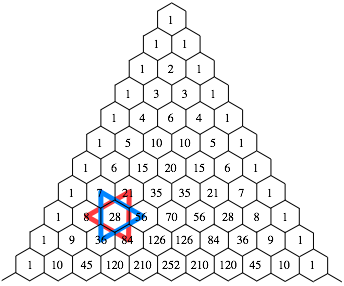Fig. 1: Star of David

Now the product of the binomial coefficients in the vertices of the blue triangle is equal to the product of the binomial coefficients in the vertices of the red triangle: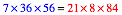General:Proof: Use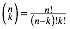and compute.

# 2        Extended Stars

Figure 2 shows an extended version of the Star of David.Fig. 2: Extended Star of David

Again we have:Figure 3 shows another example.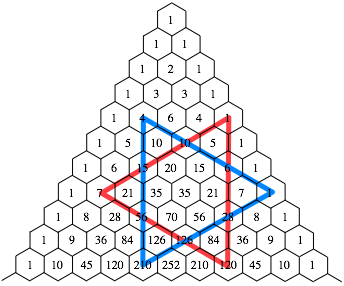Fig. 3: Another Star of David

In this example we get: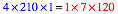General: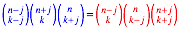Proof: Useand compute.

# 3        Rotated Stars?

If we rotate the Star of David, the Theorem does not work any more (Fig. 4).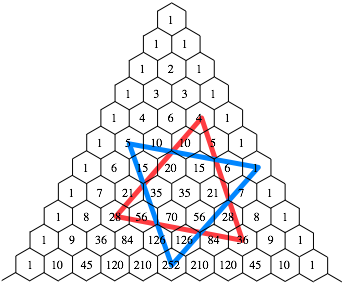Fig. 4: Rotated Star of David

We have: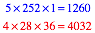Figure 5 shows a more symmetric situation of the Star of David, but the Theorem does not hold even in this case.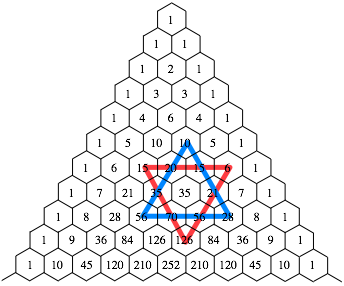Fig. 5: Symmetric position of the Star of David

We have in this case: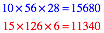# 4        Rotated triangles

We rotate the two equilateral triangles in different directions about the same amount (Fig. 6).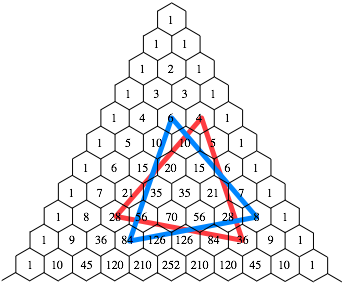Fig. 6: Rotated triangles

Now we get:General: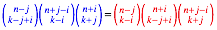Proof: Useand compute.

References

Hilton, Peter / Holton, Derek / Pedersen, Jean (1998): Mathematical Reflections: In a Room with Many Mirrors. 2nd printing. New York: Springer 1998. ISBN 0-387-94770-1.

Hilton, Peter / Holton, Derek / Pedersen, Jean (2002): Mathematical Vistas: From a Room with Many Mirrors. New York: Springer 2002. ISBN 0-387-95064-8.

Hilton, Peter and Pedersen, Jean (2010): Stop-sign theorems and binomial coefficients. The Mathematical Gazette, 94, 247-261.

Hilton, Peter and Pedersen, Jean and Séquin Carlo H. (2012): Star Theorem Patterns Relation to 2n-gons in Pascal’s Triangle — and More. Southeast Asian Bulletin of Mathematics, 36, 209-232.